Bihar Board 12th Chemistry Objective Questions and Answers

## Bihar Board 12th Chemistry Objective Answers Chapter 3 Electrochemistry

Question 1.
Which of the following is the correct cell representation for the given cell reaction ?
Zn + H2SO4→ ZnSO4 + H2
(a) Zn|Zn2+||H + |H2
(b) Zn|Zn2+||H + ,H2|Pt
(c) Zn |ZnSO4| |H2SO4| Zn
(d) Zn |H2SO4||ZnSO4| H2
(b) Zn|Zn2+||H + ,H2|Pt

Question 2.
The cell reaction of the galvanic cell: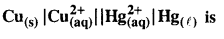(a) Hg + Cu2+→ Hg2++Cu
(b) Hg + Cu2+→ Cu+Hg+
(c) Cu + Hg → CuHg
(d) Cu + Hg2+→ Cu2+ Hg
(d) Cu + Hg2+→ Cu2+ HgQuestion 3.
Which of the following reaction is possible at anode ?
(a) 2Cr3+ +7H2O → Cr2O72- + 14H+
(b) F2 → 2F
(c) (1/2)O2 +2H+ → H2O
(d) None of these.
(a) 2Cr3+ +7H2O → Cr2O72- + 14H+

Question 4.
In the cell, |Zn2+| |Cu2+|Cu, the negative terminals ………..
(a) Cu
(b) Cu2+
(c) Zn
(d) Zn2+
(c) Zn

Question 5.
In a cell reaction,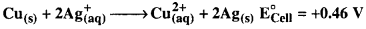If the concentration of Cu2+ ions is doubled then EºCell will be
(a) doubled
(b) halved
(c) increased by four times
(d) unchanged
(d) unchangedQuestion 6.
A standard hydrogen electrode that a zero potential because ……………..
(a) hydrogen can be most’easily oxidised
(b) hydrogen has only one electron
(c) the electrode potential is assumed to be zero
(d) hydrogen is the lightest element.
(c) the electrode potential is assumed to be zero

Question 7.
Which of the following is the correct order in which metals displace each other from the salt solution of their salts  ?
(a) Zn, Al, Mg, Fe, Cu
(b) Cu, Fe, Mg, Al, Zn
(c) Mg, Al, Zn, Fe, Cu
(d) Al, Mg, Fe, Cu, Zn
(d) Al, Mg, Fe, Cu, Zn

Question 8.
Fluorine is the best oxidising agent because it has ………..
(a) highest electron affinity
(b) highest reduction potential
(c) highest oxidation potential
(d) lowest electron affinity.
(b) highest reduction potential

Question 9.
At 25°C, Nemst equation is …………..(a)Question 10.
The correct Nernst equation for the given cell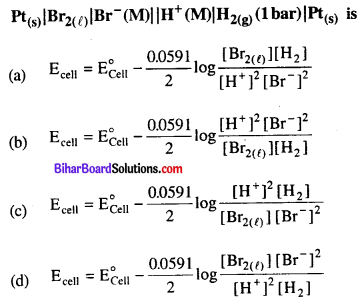Question 11.
What will be the emf of the following concentration cell at 25°C ?
Ag(s)| AgNO3(0.01M)| | AgNO3(0.05M)| Ag(s)
(a) 0.828 V
(b) 0.0413 V
(c) -0.0413 V
(d) -0.828 V
(b) 0.0413 V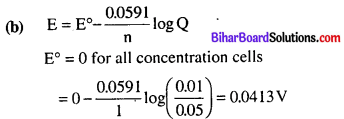Question 12.
What will be the reduction potential for the following half-cell reaction at 298 K ? [Given: [Ag+] = 0.1 M and
e„ = +0.80 V)] ………….
(a) 0.741V
(b) 0.80 V
(c) -0.80 V
(d) -0.741V
(a) 0.741V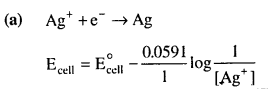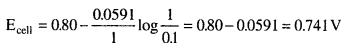Question 13.
For the cell reaction: 2Cu(aq) → Cu(s) + Cu2+ the standard ceil potential is 0.36 V. The equilibrium constant for the reaction is ………….
(a) 1.2 x 106
(b) 7.4 x 1012
(c) 2.4 x 106
(d) 5.5 x 108
(a) 1.2 x 106

Question 14.
cell for the reaction, 2H2O+ H3O+ + OH” at 25°C is -0.8277 V. The equilibrium constant for the reaction is ………….
(a) 10-14
(b) 10-23
(c) 10-7
(d) 10-21
(a) 10-14

Question 15.
Cell reaction is spontaneous, when …………….
(a) E°d is negative
(b) ΔG° is negative
(c) E°oxid is positive
(d) ΔG° is positive
(b) ΔG° is negative

Question 16.
ΔG° f°r the reaction, Cu2++ Fe → Fe2+ + Cu is(a) 11.44 kJ
(b) 180.8 kJ
(c) 150.5 kJ
(d) 28.5 kJ
(c) 150.5 kJ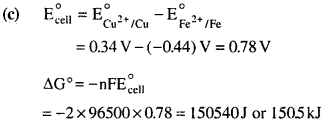Question 17.
Units of the properties measured are given below. Which of the properties has not been matched correctly ?
(a) Molar conductance = S m2 mol-1
(b) Cell constant = m-1
(c) Specific conductance = S m2
(d) Equivalent conductance = S m2 (g eq)-1
(c) Specific conductance = S m2

Question 18.
Molar conductivity of 0.15 M solution of KCl at 298 K, if its conductivity is 0.0152 S cm-1 will be
(a) 124 Ω-1 cm2    mol-1
(b) 204  Ω-1 cm2 mol-1
(c) 101 Ω-1 cm2 mol-1
(d) 300  Ω-1 cm2 mol-1
(c) 101 Ω-1 cm2   mol-1

Question 19.
The molar conductivity is maximum for the solution of concentration
(a) 0.004 M
(b) 0.002 M
(c) 0.005 M
(d) 0.001 M
(d) 0.001 M

Question 20.
Specific conductance of 0.1 M NaCl solution is 1.01 x 10-2 ohm-1 cm-1. Its molar conductance in ohm-1 cm2 mol-1
(a) 1.01 x 102
(b) 1.01 x 103
(c) 1.01 x 104
(d) 1.01
(a) 1.01 x 102

Question 21.
When water is added to an aqueous solution of an electrolyte, what is the change in specific conductivity of the electrolyte ?
(a) Conductivity decreases
(b) Conductivity increases
(c) Conductivity remain same
(d) Conductivity does not depend on number of ions
(a) Conductivity decreasesQuestion 22.
Mark the correct choice of electrolytes represented in the graph.(a) A → 4 NH4OH, B → NaCl
(b) A →NH4OH,B → NH4Cl
(c) A → CH3COOH, B → 4CH3COONa
(d) A → KCl, B → 4 NH4OH
(d) A → KCl, B → 4 NH4OH

Question 23.
Molar conductivity of NH4OH can be calculated by the equation,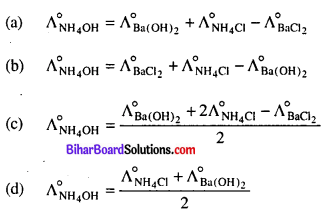(c)

Question 24.
Limiting molar conductivity of NaBr is
(a) Δ°mNaBr = Δ°mNaCl + Δ°mKBr
(b) Δ°mNaBr = Δ°mNaCl + Δ°mKBr – Δ°mKCl
(c) Δ°mNaBr = Δ°mNaOH + Δ°mNaBr – Δ°mNaCl
(d) Δ°mNaBr = Δ°mNaCl – Δ°mNaBr
(b) Δ°mNaBr = Δ°mNaCl + Δ°mKBr – Δ°mKCl

Question 25.
Δ°m(NH4OH) is equal to …………..
(a) Δ°m (NH4OH) + Δ°m (NH4a) –  -Δ°m(HCl)
(b) Δ°m(NH4Cl) + Δ°m (NaOH) – Δ°m(Nao)
(c) Δ°m(NH4Cl) + Δ°m(NaCl) –  Δ°m(NaOH)
(d) Δ°m(NaOH) +Δ°m(NaCI) – Δ°m(NH4Cl)
(b) Δ°m(NH4Cl) + Δ°m (NaOH) – Δ°m(Naa)

Question 26.
In an electrolytic cell, the flow of electrons is
(a) from cathode to anode in the solution
(b) from cathode to anode through external supply
(c) from cathode to anode through internal supply
(d) from anode to cathode through internal supply.
(c) from cathode to anode through internal supplyQuestion 27.
How long would it take to deposit 50 g of Ai from an electrolytic cell containing Al2O3 using a current of 105 ampere
(a) 54 h
(b) 1.42 h
(c) 32 h
(d) 2.15 h
(b) 1.42 h

Question 28.
The charge required for reducing 1 mole of MnO4 to Mn2+ is ……………….
(a) 93 x 105 C
(b) 2.895 x  105 C
(c) 4.28 x  105 C
(d) 4.825 x  105 C
(d) 4.825 x  105 C

Question 29.
How much electricity in terms of Faraday is required to  produce 100 g of Ca from molten CaCl2 ?
(a) IF
(b) 2F
(c) 3 F
(d) 5F
(a) IF

Question 30.
How many coulombs of electricity is required to reduce 1 mole of Cr2O2 in acidic medium ?
(a) 4 x  96500C
(b) 6 x  96500 C
(c) 2 x 96500 C
(d) 1 x  96500 C
(b) 6 x  96500 C

Question 31.
How much metal will be deposited when a current of 12 ampere with 75% efficiency is passed through the cell for 3 h ? (Given : Z = 4 x 10-4)
(a) 32.4 g
(b) 38.8 g
(c) 0 g
(d) 22.4 g
(b) 38.8 g

Question 32.
How many moles of Pt may be deposited on the cathode when 0.80 F of electricity is passed through a 1.0 M solution of Pt4+ ?
(a) 0.1 mol
(b) 0.2  mol
(c) 4 mol
(d) 0.6  mol
(b) 0.2  mol
(b) Pt4+ + 4e → Pt
4 moles of electricity or 4 F of electricity is required to deposit 1 mole of Pt.
0. 80 F of electricity will deposit
$$\frac{1}{4}$$ 0.80 = 0.20 molQuestion 33.
If 54 g of silver is deposited during an electrolysis reaction, how much aluminium will be deposited by the same amount of electric current ?
(a) 2.7 g
(b) 4.5  g
(c) 27 g
(d) 5.4  g
(b) 4.5gQuestion 34.
During the electrolysis of dilute sulphuric acid, the following process is possible at anode.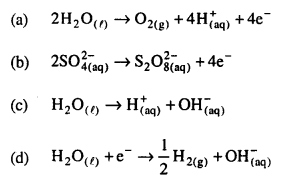(a)

Question 35.
When a lead storage battery is discharged,
(b) oxygen gas is evolvedQuestion 36.
The reaction which is taking place in nickel-cadmium battery can be represented by which of the following equation
(a) Cd + NiO2 + 2H2O → 4 Cd(OH)2 + Ni(OH)2
(b) Cd + NiO2 + 2 OH+ → Ni + Cd(OH)2
(c) Ni + Cd(OH)2 → Cd + Ni(OH)2
(d) Ni(OH)2 +Cd(OH)2 → Ni + Cd + 2H2O
(a) Cd + NiO2 + 2H2O → 4 Cd(OH)2 + Ni(OH)2

Question 37.
Which of the following reactions does not take place during rusting ?
(a) 42 CO3 2H+ + CO+
(b) 4Fe2+ + O2(dry) → Fe2O3
(c) 4Fe2+ +O2 +4H2O → 2Fe2O3 +8H+
(d) Fe2O3 + xH2O → Fe2O3. x H2O
(a) 42 CO3 2H+ + CO+

Question 38.
Which of the following reactions does not take place during rusting ?
(a)(b) 4Fe2+ + O2(dry) → Fe2O3
(c) 4Fe2+ + O2 +4H2O → 2Fe2O3 +8H+
(d) Fe2O3 + xH2O → Fe2O3. xH2O
(b) 4Fe2+ + O2(dry) → Fe2O3

Question 39.
The difference between the electrode potentials of two electrodes when no current is drawn through the cell is called
(a) cell potential
(b) cell emf
(c) potential difference
(d) cell voltage
(b) cell emfQuestion 40.
An electrochemical cell can behave like an electrolytic cell when ___
(a) Ecell = 0
(b) Ecell > Eext
(c) Eextl > Ecell
(d) Ecell = Eext
(c) Eextl > Ecell

Question 41.
Use the data given in Q. 61 and find out the most stable ion in its reduced form.
(a) Cl-
(b) Cr3+
(c) Cr
(d) Mn2+
(d) Mn2+

Question 42.
Use the data of Q. 61 and find out the most stable oxidised species…….
(a) Cr3+
(b) MnO4
(c) Cr2O2-
(d) Mn2+
(a) Cr3+

Question 43.
The quantity of charge required to obtain one mole of aluminium from Al2O3 is____________
(a) 1F
(b) 6F
(c) 3F
(d) 2F
(c) 3F

Question 44.
The cell constant of a conductivity cell_____________
(a) changes with change of electrolyte
(b) changes with change of concentration of electrolyte
(c) changes with temperature of electrolyte
(d) remains constant for a cell